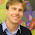Thursday, November 12, 2009

Stokes Law

I like Stoke's Law. It's one of those fundamental relationships that is very powerfull:

A particle, falling through a fluid under its own weight. There are two fundamental forces at work: gravity, which tends to accelerate the particle downward, and an opposing drag force, which is a result of the density of the fluid through which the particle falls. As the particle falls downwards, it will accelerate due to gravity until the drag force (which is velocity dependent) equals the gravitational force. At the point the two forces are equal, there is no more acceleration, and the particle continues downward at a constant speed (uniform motion). This is the object's terminal velocity. It's the basic principle upon which parachutes are based. It also explains why a penny dropped from the top of the Empire State Building won't bury itself into the concrete below. The drag of the fluid (air in this case) balances out gravity, and the object falls at a constant speed.

This same principle applies to sediments deposited in air or water - they fall through a column of water at a constant velocity. The equation that describes the drag force (FD) on a sphere is:

FD = CDAρvs2/2

Where CD is the drag coefficient, A is the cross-sectional area of the sphere (A=πr2), ρ is the density of the fluid, and vs is its velocity. The gravitational force (FW) acting on the sphere - its submerged weight - is described by:

FW = VΔρg

Where V is the volume of the sphere (4πr3)/3, Δρ is the difference in density between the sphere and the fluid, and g is the acceleration due to gravity (9.8 m/s2. For uniform motion, then, FW = FD. And if we know the density and size of the sphere, the density of water, gravitational acceleration, and drag, we could estimate how long it would take this particle to fall through a column of water.

Now it would be tempting to calculate the settling velocity for a tiny sand grain, or clay particle and then infer a time required to deposit a given thickness of sedimentary rock from this. The real world is not quite as simple as this. However, a nearly spherical grain 0.01 mm in diameter (a silt-sized particle) falling through non-turbulent water would reach a terminal velocity of about 0.01 cm per second. Thus, to fall 200 m (the average depth of the continental shelf), the grain would take about 2,000,000 seconds (20,000 cm/0.01 cm s-1). That's more than 23 days.

Now it's also tempting to calculate the settling velocity for a clay-sized particle (it would take about two orders of magnitude longer to fall 200 m (~230 days), but - as I've said before - the real world is a little more complicated. But, a flood model would do well to account for Stoke's Law: for a global flood would create a great deal of turbulence, and while some super-concentrated muds may settle quickly, some mud would remain suspended for much longer. As Noah supposedly waited for the Dove to return - both times - this mud would remain suspended far longer than the sands and gravels and everything else that supposedly was deposited in this world-wide event. Thus, at the very end of this "flood," a thin drape of mud should cover much of the world (those of you who have seen the effects of flooded rivers (or flooded basements) know this well. And yet, we do not see any evidence for a world-wide mud drape anywhere! While I can understand the desire of YEFPs to reconcile the natural world with their biblical beliefs, it just ain't gonna happen. Ever.

Those that attempt to explain the world's geology as a supernaturally-caused event MUST violate the physical constants that control our world. Simple things like gravity. And heat. Once you claim that physical constants are not constant, the laws of physics cannot be applied to anything. So those that use the laws of physics to justify violating those same laws are caught in a self-nullifying situation. Catch-22. Uniformitarianism works because it cannot violate the laws of physics. Ever.

1.2.3.4.